/

### Derivative Pricing Theory: Optimising a trinomial lattice to converge more rapidly than a binomial lattice

In a situation where,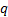and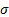are constant through time, a trinomial lattice framework that seems to provide effective enhancements in convergence properties is as set out in Kemp (1997). Suppose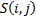characterises the lattice point in which we have hadtime steps from outset and we aresteps above the lowest possible that is included in the trinomial tree. We propose a lattice calculated as follows. Movement should be allowed fromto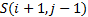(i.e. up,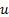),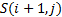(sideways,) or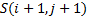(down,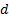), with the nodes of the lattice defined by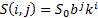for some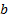and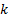defined as below. The backwards equation is then defined as follows: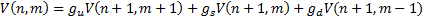where: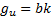,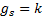,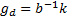,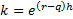,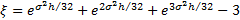,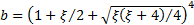and additionally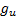,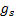and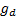are chosen so that the following three simultaneous equations are satisfied: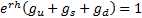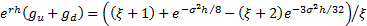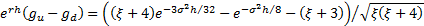Such a lattice is designed so that if we were to price a derivative paying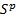for each of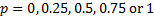, then the lattice would give exactly the right answer.  For standard types of European and American options this calibrated trinomial lattice can converge much more rapidly than the equivalent binomial lattice. If,andchange through time (in a deterministic way) then corresponding lattices (including risk-neutral lattice migration probabilities) can be derived in an analogous fashion with the same end goal, but the formulae involved will be time-varying and therefore more complicated.

The complexity of the above highlights an important practical aspect of derivative pricing. Whilst the principles are in some sense conceptually relatively easy to grasp, the mathematics can get complicated very quickly as soon as you start trying to apply the concepts in practice, particularly if you want to do so in ways that are relatively efficient to compute. It is not always clear how complex it might be best to get in such a quest. For example:

(a)    We might expect to be able to improve convergence still further by using quadrinomial or even more complicated lattices, but the effort involved in programming them becomes progressively more complicated, and it becomes less easy to ensure that the optimal lattice structure is recombining, so for fast numerical computation of options using lattices, trinomial lattices are likely to be preferred over more complex lattices in most circumstances.

(b)   There are actually two main sorts of errors arising with lattice pricing methods.  The first sort of error relates to propagation errors via the backwards equation, which can be much reduced by using trinomial lattices rather than binomial ones.  The second sort of error involves the approximation of a continuous pay-off at maturity with one involving discrete amounts at each maturity node.  It can be reduced by setting the pay-off at each maturity node equal to the average of maturity pay-off for prices of the underlying closest to the node.  This second sort of error is not improved merely by use of a trinomial lattice. Typically better still is to use semi-analytic lattice integrator approaches, see e.g. Hu, Kerkhof, McCloud and Wackertapp (2007) or Semi-analytic lattice integrator approaches, if the payoff function can be approximated by piecewise polynomials and the risk-neutral probability distribution is analytically tractable.

(c)    Other ways exist of improving convergence of binomial lattices not (directly) involving trinomial lattices or the like. Because the pricing formulae can be rewritten as expectations (under the risk neutral probability distribution) and because expectations are merely specific instances of the mathematical process of integration, lattices can be thought of as approximate ways of carrying out integration. Various mathematical tools and techniques exist that can be applied to this task, see e.g. Press et al. (2007). Some of these tools appear to be as good as if not better than using trinomial lattices (and even if they are not actually better they may be rather simpler to understand and program reliably).